# Common Core: 7th Grade Math : Develop and Compare Probability Models and Find Probabilities of Events: CCSS.Math.Content.7.SP.C.7

## Example Questions

### Example Question #861 : Grade 7

If John were to roll a die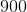times, roughly how many times would he roll aor a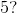Explanation:

A die hassides, with each side displaying a number between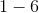Let's first determine the probability of rolling aor aafter John rolls the die a single time.

There is a total ofsides on a die and we have one value ofand one value of; thus, our probability is: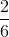This means that roughlyof John's rolls will be aor a; therefore, in order to calculate the probability we can multiplyby—the number of times John rolls the die.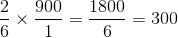If John rolls a dietimes, then he will roll aor aroughlytimes.

### Example Question #1 : Develop And Compare Probability Models And Find Probabilities Of Events: Ccss.Math.Content.7.Sp.C.7

If John were to roll a dietimes, roughly how many times would he roll a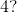Explanation:

A die hassides, with each side displaying a number betweenLet's first determine the probability of rolling aafter John rolls the die a single time.

There is a total ofsides on a die and only one value ofon one side; thus, our probability is:This means that roughlyof John's rolls will be a; therefore, in order to calculate the probability we can multiplyby—the number of times John rolls the die.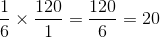If John rolls a dietimes, then he will roll aroughlytimes.

### Example Question #1 : Develop And Compare Probability Models And Find Probabilities Of Events: Ccss.Math.Content.7.Sp.C.7

If John were to roll a die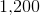times, roughly how many times would he roll a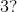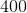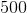Explanation:

A die hassides, with each side displaying a number betweenLet's first determine the probability of rolling aafter John rolls the die a single time.

There is a total ofsides on a die and only one value ofon one side; thus, our probability is:This means that roughlyof John's rolls will be a; therefore, in order to calculate the probability we can multiplyby—the number of times John rolls the die.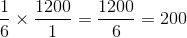If John rolls a dietimes, then he will roll aroughlytimes.

### All Common Core: 7th Grade Math Resources